# 绘制几何形状¶

## 三大类形状绘制函数¶

• 只绘制形状轮廓线的函数。这类函数用’xxx()’ 的形式命名(“xxx”是所绘制形状的名称，如circle)。下面的程序绘制了一个边线为蓝色的圆。
```from easygraphics import *

def main():
init_graph(200, 150)
set_color(Color.BLUE)
set_fill_color(Color.RED)
circle(100, 75, 60)
pause()
close_graph()

easy_run(main)
```
• 同时绘制形状轮廓并填充形状内部的函数。这类函数用’draw_xxx()’ 的形式命名(“xxx”是所绘制形状的名称，如draw_circle)。下面的程序绘制了一个边线为蓝色内部为红色的圆。
```from easygraphics import *

def main():
init_graph(200, 150)
set_color(Color.BLUE)
set_fill_color(Color.RED)
draw_circle(100, 75, 60)
pause()
close_graph()

easy_run(main)
```
• 不绘制形状轮廓线，只填充形状内部的函数。这类函数用’fill_xxx()’ 形式命名(“xxx”是所绘制形状的名称，如fill_circle)。下面的程序绘制（填充）了一个无边线内部为红色的圆。
```from easygraphics import *

def main():
init_graph(200, 150)
set_color(Color.BLUE)
set_fill_color(Color.RED)
fill_circle(100, 75, 60)
pause()
close_graph()

easy_run(main)
```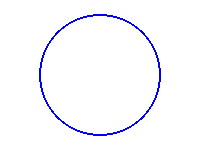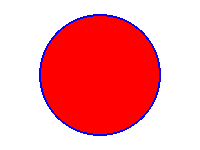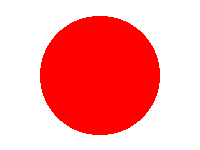circle() draw_circle() fill_circle()

## 角¶

```from easygraphics import *

def main():
init_graph(400, 300)
set_color("red")
set_fill_color("lightyellow")
draw_pie(200, 150, 45, 135, 100, 70)
pause()
close_graph()

easy_run(main)
```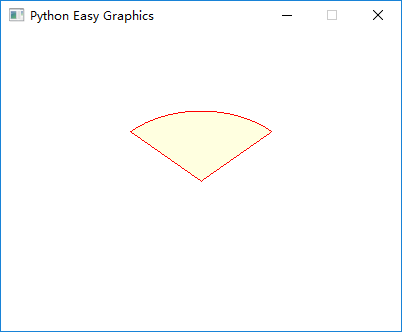## 多边形(Polygon)¶

polygon()/draw_polygon()/fill_polygon()需要提供一个list（列表）参数，其中存放着各顶点的位置。在列表中，每个顶点用两个数来表示（其x和y坐标）。所以，如果有n个顶点，那么列表中应该有2n个数。

```from easygraphics import *

def main():
init_graph(400, 300)
set_color(Color.DARK_BLUE)
set_fill_color(Color.LIGHT_MAGENTA)
draw_polygon(50, 50, 350, 250, 50, 150)
pause()
close_graph()

easy_run(main)
```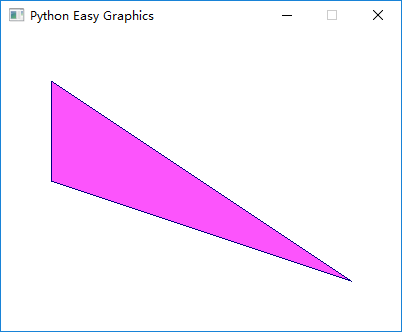## 折线（Polylines）¶poly_line() and draw_poly_line()需要提供一个list（列表）参数，其中存放着各端点的位置。在列表中，每个端点用两个数来表示（其x和y坐标）。所以n个端点就需要2n个数来表示。

```from easygraphics import *

def main():
init_graph(400, 300)
draw_poly_line(50, 50, 350, 75, 50, 150, 350, 175, 50, 250, 350, 275)
pause()
close_graph()

easy_run(main)
```## 贝塞尔曲线（Bézier curve）¶```from easygraphics import *

def main():
init_graph(600, 400)
points = [300, 50, 200, 50, 200, 200, 100, 200]
draw_bezier(*points)
pause()
close_graph()

easy_run(main)
```

```from easygraphics import *

def main():
init_graph(600, 400)
points = [300, 50, 200, 50, 100, 200]
pause()
close_graph()

easy_run(main)
```

## 泛洪填充（Flood Fill）¶

```from easygraphics import *

def main():
init_graph(400, 200)
arc(200, -40, 180, 360, 220, 220)
arc(200, 240, 0, 180, 220, 220)
set_fill_color(Color.DARK_RED)
flood_fill(200, 100, Color.BLACK)
pause()
close_graph()

easy_run(main)
```## 填充规则（Fill Rule）¶```from easygraphics import *

def main():
init_graph(420, 200)

set_fill_rule(FillRule.ODD_EVEN_FILL)
set_fill_color("lightgray")
draw_polygon(50, 50, 150, 50, 60, 110, 100, 20, 140,110,50,50)

draw_text(5,150,"FillRule.ODD_EVEN_FILL")

translate(220,0)

set_fill_rule(FillRule.WINDING_FILL)
set_fill_color("lightgray")
draw_polygon(50, 50, 150, 50, 60, 110, 100, 20, 140,110,50,50)

draw_text(5,150,"FillRule.WINDING_FILL")

pause()
close_graph()

easy_run(main)
```

## 高级绘图¶

EasyGraphics支持绘制所有的基本图形：点、线、圆、椭圆（弧、扇形、弓形）、矩形、多边形、圆角矩形等。如果需要更高级的绘制功能，可以通过 Image 对象的成员方法get_painter()来获取内部的 QPainter 对象，然后直接用它来进行绘制。